# Linear Computational Circuitry

• #### Question 1

 Don’t just sit there! Build something!!

Learning to mathematically analyze circuits requires much study and practice. Typically, students practice by working through lots of sample problems and checking their answers against those provided by the textbook or the instructor. While this is good, there is a much better way.

You will learn much more by actually building and analyzing real circuits, letting your test equipment provide the “answers” instead of a book or another person. For successful circuit-building exercises, follow these steps:

1. Carefully measure and record all component values prior to circuit construction.
2. Draw the schematic diagram for the circuit to be analyzed.
3. Carefully build this circuit on a breadboard or other convenient medium.
4. Check the accuracy of the circuit’s construction, following each wire to each connection point, and verifying these elements one-by-one on the diagram.
5. Mathematically analyze the circuit, solving for all voltage and current values.
6. Carefully measure all voltages and currents, to verify the accuracy of your analysis.
7. If there are any substantial errors (greater than a few percent), carefully check your circuit’s construction against the diagram, then carefully re-calculate the values and re-measure.

Avoid using the model 741 op-amp, unless you want to challenge your circuit design skills. There are more versatile op-amp models commonly available for the beginner. I recommend the LM324 for DC and low-frequency AC circuits, and the TL082 for AC projects involving audio or higher frequencies.

As usual, avoid very high and very low resistor values, to avoid measurement errors caused by meter “loading”. I recommend resistor values between 1 kΩ and 100 kΩ.

One way you can save time and reduce the possibility of error is to begin with a very simple circuit and incrementally add components to increase its complexity after each analysis, rather than building a whole new circuit for each practice problem. Another time-saving technique is to re-use the same components in a variety of different circuit configurations. This way, you won’t have to measure any component’s value more than once.

• #### Question 2

Suppose I set a potentiometer to the 30% position, so that the wiper is 30% of the way up from the bottom:Describe the mathematical relationship between Vin and Vout with the potentiometer in this position. What does this suggest about the usefulness of a potentiometer as an analog computational element?

• #### Question 3

What historical significance does the name “operational amplifier” have? Of course, it should be obvious that these devices are amplifiers, but what is meant by the word operational?

• #### Question 4

Write a mathematical expression in the form of y = ... x describing the function of this circuit:• #### Question 5

Write a mathematical expression in the form of y = ... x describing the function of this circuit: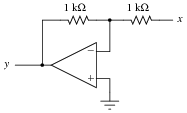• #### Question 6

Ideally, an inverting amplifier circuit may be comprised of just one op-amp and two resistors, as such:However, if high accuracy is desired, a third resistor must be added to the circuit, in series with the other op-amp input: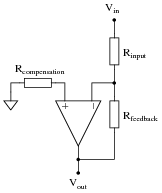Explain what this “compensation” resistor is compensating for, and also what its value should be.

• #### Question 7

We know that an opamp connected to a voltage divider with a voltage division ratio of $$\frac{1}{2}$$ will have an overall voltage gain of 2, and that the same circuit with a voltage division ratio of $$\frac{2}{3}$$ will have an overall voltage gain of 1.5, or $$\frac{3}{2}$$:There is definitely a mathematical pattern at work in these non-inverting opamp circuits: the overall voltage gain of the circuit is the mathematical inverse of the feedback network’s voltage gain.

Building on this concept, what do you think would be the overall function of the following opamp circuits?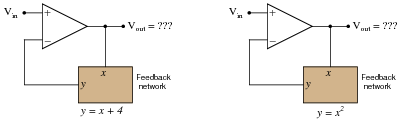• #### Question 8

The simple resistor network shown here is known as a passive averager. Describe what the word “passive” means in this context, and write an equation describing the output voltage (Vd) in terms of the input voltages (Va, Vb, and Vc):Hint: there is a network theorem that directly applies to this form of circuit, and it is known as Millman’s Theorem. Research this theorem and use it to generate your equation!

• #### Question 9

Add an op-amp circuit to the output of this passive averager network to produce a summer circuit: an operational circuit generating an output voltage equal to the sum of the four input voltages. Then, write an equation describing the whole circuit’s function.• #### Question 10

Write a mathematical equation for this op-amp circuit, assuming all resistor values are equal:What is this circuit typically called?

• #### Question 11

This opamp circuit is known as a difference amplifier, sometimes called a subtractor. Assuming that all resistor values are equal in the circuit, write an equation expressing the output (y) as a function of the two input voltages (a and b):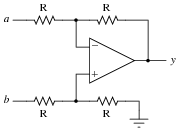• #### Question 12

Re-write the equation for this differential amplifier circuit, assuming that the marked resistor fails open: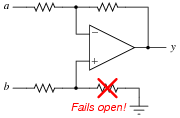• #### Question 13

Write a mathematical expression in the form of y = ... x describing the function of this circuit: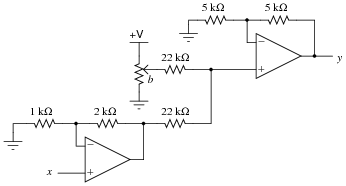• #### Question 14

How much current will go through the load in this op-amp circuit?What impact will a change in load resistance have on the operation of this circuit? What will change, if anything, supposing the load resistance were to increase?

• #### Question 15

How much current will go “through” the capacitor in this op-amp circuit, and what effect does this have on the output voltage?• #### Question 16

What will the output voltage of this integrator circuit do when the DPDT (“Double-Pole, Double-Throw”) switch is flipped back and forth?Be as specific as you can in your answer, explaining what happens in the switch’s “up” position as well as in its “down” position.

• #### Question 17

Calculate the output voltage rate-of-change ([dv/dt]) for this active integrator circuit, being sure to explain all the steps involved in determining the answer:• #### Question 18

Calculate the input voltage needed to produce an output voltage rate-of-change $$\frac{dv}{dt}$$ of -25 volts per second in this active integrator circuit: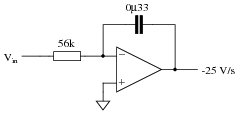• #### Question 19

The purpose of this circuit is to provide a pushbutton-adjustable voltage. Pressing one button causes the output voltage to increase, while pressing the other button causes the output voltage to decrease. When neither button is pressed, the voltage remains stable. Essentially, it is an active integrator circuit, with resistors R1 and R2 and capacitor C1 setting the rates of integration:After working just fine for quite a long while, the circuit suddenly fails: now it only outputs zero volts DC all the time. Identify at least one component or wiring failure that would account for the circuit’s faulty behavior, and explain why that fault would cause the circuit to act like this.

• #### Question 20

Integrator circuits may be understood in terms of their response to DC input signals: if an integrator receives a steady, unchanging DC input voltage signal, it will output a voltage that changes with a steady rate over time. The rate of the changing output voltage is directly proportional to the magnitude of the input voltage: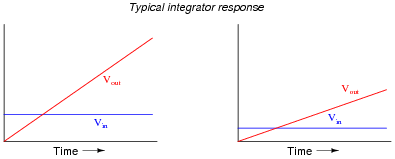A symbolic way of expressing this input/output relationship is by using the concept of the derivative in calculus (a rate of change of one variable compared to another). For an integrator circuit, the rate of output voltage change over time is proportional to the input voltage:

 dVout dt ∝ Vin

A more sophisticated way of saying this is, “The time-derivative of output voltage is proportional to the input voltage in an integrator circuit.” However, in calculus there is a special symbol used to express this same relationship in reverse terms: expressing the output voltage as a function of the input. For an integrator circuit, this special symbol is called the integration symbol, and it looks like an elongated letter “S”:

 Vout ∝ ⌠ ⌡ T 0 Vin dt

Here, we would say that output voltage is proportional to the time-integral of the input voltage, accumulated over a period of time from time=0 to some point in time we call T.

“This is all very interesting,” you say, “but what does this have to do with anything in real life?” Well, there are actually a great deal of applications where physical quantities are related to each other by time-derivatives and time-integrals. Take this water tank, for example: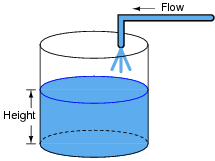One of these variables (either height H or flow F, I’m not saying yet!) is the time-integral of the other, just as Vout is the time-integral of Vin in an integrator circuit. What this means is that we could electrically measure one of these two variables in the water tank system (either height or flow) so that it becomes represented as a voltage, then send that voltage signal to an integrator and have the output of the integrator derive the other variable in the system without having to measure it!

Your task is to determine which variable in the water tank scenario would have to be measured so we could electronically predict the other variable using an integrator circuit.

• #### Question 21

We know that the output of an integrator circuit is proportional to the time-integral of the input voltage:Vout ∝ ⌠ ⌡ T 0 Vin dt

But how do we turn this proportionality into an exact equality, so that it accounts for the values of R and C? Although the answer to this question is easy enough to simply look up in an electronics reference book, it would be great to actually derive the exact equation from your knowledge of electronic component behaviors! Here are a couple of hints:

 I = V R i = C dv dt

• #### Question 22

If an object moves in a straight line, such as an automobile traveling down a straight road, there are three common measurements we may apply to it: position (x), velocity (v), and acceleration (a). Position, of course, is nothing more than a measure of how far the object has traveled from its starting point. Velocity is a measure of how fast its position is changing over time. Acceleration is a measure of how fast the velocity is changing over time.

These three measurements are excellent illustrations of calculus in action. Whenever we speak of “rates of change,” we are really referring to what mathematicians call derivatives. Thus, when we say that velocity (v) is a measure of how fast the object’s position (x) is changing over time, what we are really saying is that velocity is the “time-derivative” of position. Symbolically, we would express this using the following notation:

 v = dx dt

Likewise, if acceleration (a) is a measure of how fast the object’s velocity (v) is changing over time, we could use the same notation and say that acceleration is the time-derivative of velocity:

 a = dv dt

Since it took two differentiations to get from position to acceleration, we could also say that acceleration is the second time-derivative of position:

 a = d2x dt2

“What has this got to do with electronics,” you ask? Quite a bit! Suppose we were to measure the velocity of an automobile using a tachogenerator sensor connected to one of the wheels: the faster the wheel turns, the more DC voltage is output by the generator, so that voltage becomes a direct representation of velocity. Now we send this voltage signal to the input of a differentiator circuit, which performs the time-differentiation function on that signal. What would the output of this differentiator circuit then represent with respect to the automobile, position or acceleration? What practical use do you see for such a circuit?

Now suppose we send the same tachogenerator voltage signal (representing the automobile’s velocity) to the input of an integrator circuit, which performs the time-integration function on that signal (which is the mathematical inverse of differentiation, just as multiplication is the mathematical inverse of division). What would the output of this integrator then represent with respect to the automobile, position or acceleration? What practical use do you see for such a circuit?

• #### Question 23

A familiar context in which to apply and understand basic principles of calculus is the motion of an object, in terms of position (x), velocity (v), and acceleration (a). We know that velocity is the time-derivative of position (v = $$\frac{dx}{dt}$$) and that acceleration is the time-derivative of velocity (a = $$\frac{dv}{dt}$$). Another way of saying this is that velocity is the rate of position change over time, and that acceleration is the rate of velocity change over time.

It is easy to construct circuits which input a voltage signal and output either the time-derivative or the time-integral (the opposite of the derivative) of that input signal. We call these circuits “differentiators” and ïntegrators,” respectively.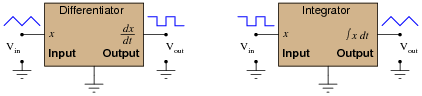Integrator and differentiator circuits are highly useful for motion signal processing, because they allow us to take voltage signals from motion sensors and convert them into signals representing other motion variables. For each of the following cases, determine whether we would need to use an integrator circuit or a differentiator circuit to convert the first type of motion signal into the second:

Converting velocity signal to position signal: (integrator or differentiator?)
Converting acceleration signal to velocity signal: (integrator or differentiator?)
Converting position signal to velocity signal: (integrator or differentiator?)
Converting velocity signal to acceleration signal: (integrator or differentiator?)
Converting acceleration signal to position signal: (integrator or differentiator?)

Also, draw the schematic diagrams for these two different circuits.

• #### Question 24

This active integrator circuit processes the voltage signal from an accelerometer, a device that outputs a DC voltage proportional to its physical acceleration. The accelerometer is being used to measure the acceleration of an athlete’s foot as he kicks a ball, and the job of the integrator is to convert that acceleration signal into a velocity signal so the researchers can record the velocity of the athlete’s foot:During the set-up for this test, a radar gun is used to check the velocity of the athlete’s foot as he does come practice kicks, and compare against the output of the integrator circuit. What the researchers find is that the integrator’s output is reading a bit low. In other words, the integrator circuit is not integrating fast enough to provide an accurate representation of foot velocity.

Determine which component(s) in the integrator circuit may have been improperly sized to cause this calibration problem. Be as specific as you can in your answer(s).

• #### Question 25

How much current (I) would have to be forced through the resistor in order to generate an output voltage of 5 volts?• #### Question 26

How much current (I) would have to be forced through the resistor in order to generate an output voltage of 5 volts?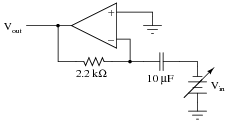At what rate would Vin have to increase in order to cause this amount of current to go “through” the capacitor, and thereby cause 5 volts to appear at the Vout terminal? What does this tell us about the behavior of this circuit?

• #### Question 27

We know that the output of a differentiator circuit is proportional to the time-derivative of the input voltage:Vout ∝ dVin dt

But how do we turn this proportionality into an exact equality, so that it accounts for the values of R and C? Although the answer to this question is easy enough to simply look up in an electronics reference book, it would be great to actually derive the exact equation from your knowledge of electronic component behaviors! Here are a couple of hints:

 I = V R i = C dv dt

• #### Question 28

You are part of a team building a rocket to carry research instruments into the high atmosphere. One of the variables needed by the on-board flight-control computer is velocity, so it can throttle engine power and achieve maximum fuel efficiency. The problem is, none of the electronic sensors on board the rocket has the ability to directly measure velocity. What is available is an altimeter, which infers the rocket’s altitude (it position away from ground) by measuring ambient air pressure; and also an accelerometer, which infers acceleration (rate-of-change of velocity) by measuring the inertial force exerted by a small mass.

The lack of a “speedometer” for the rocket may have been an engineering design oversight, but it is still your responsibility as a development technician to figure out a workable solution to the dilemma. How do you propose we obtain the electronic velocity measurement the rocket’s flight-control computer needs?

• #### Question 29

A Rogowski Coil is essentially an air-core current transformer that may be used to measure DC currents as well as AC currents. Like all current transformers, it measures the current going through whatever conductor(s) it encircles.

Normally transformers are considered AC-only devices, because electromagnetic induction requires a changing magnetic field ($$\frac{dφ}{dt}$$) to induce voltage in a conductor. The same is true for a Rogowski coil: it produces a voltage only when there is a change in the measured current. However, we may measure any current (DC or AC) using a Rogowski coil if its output signal feeds into an integrator circuit as shown:Connected as such, the output of the integrator circuit will be a direct representation of the amount of current going through the wire.

Explain why an integrator circuit is necessary to condition the Rogowski coil’s output so that output voltage truly represents conductor current.

• #### Question 30

A Rogowski coil has a mutual inductance rating of 5 μH. Calculate the size of the resistor necessary in the integrator circuit to give the integrator output a 1:1 scaling with the measured current, given a capacitor size of 4.7 nF:That is, size the resistor such that a current through the conductor changing at a rate of 1 amp per second will generate an integrator output voltage changing at a rate of 1 volt per second.

• #### Question 31

Practical integrator circuits must have a compensating resistor connected in parallel with the capacitor, in the feedback loop. Typically, this resistor value is very large: about 100 times as large as Rin.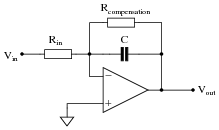Describe why this is a necessity for accurate integration. Hint: an ideal opamp would not need this resistor!

• #### Question 32

Practical op-amp integrator and differentiator circuits often cannot be built as simply as their “textbook” forms usually appear: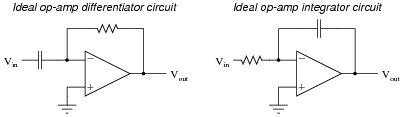The voltage gains of these circuits become extremely high at certain signal frequencies, and this may cause problems in real circuitry. A simple way to “tame” these high gains to moderate levels is to install an additional resistor in each of the circuits, as such:The purpose of each resistor is to “dominate” the impedance of the RC network as the input signal frequency approaches the point at which problems would occur in the ideal versions of the circuits. In each of these “compensated” circuits, determine whether the value of the compensation resistor needs to be large or small compared to the other resistor, and explain why.

Of course, this solution is not without problems of its own. By adding this new resistor to each circuit, a half-power (-3 dB) cutoff frequency point is created by the interaction of the compensation resistor and the capacitor, as predicted by the equation fc = [1/(2 πRcompC)]. The value predicted by this equation establishes a practical limit for the differentiation and integration functions, respectively. Operating on the wrong side of the frequency limit will result in an output waveform that is not the true time-derivative or time-integral of the input waveform. Determine whether the fc value constitutes a low frequency limit or a high frequency limit for each circuit, and explain why.

• #### Question 33

The chain rule of calculus states that:

 dx dy dy dz = dx dz

Similarly, the following mathematical principle is also true:

dx

dy
=  dx dz

 dy dz

It is very easy to build an opamp circuit that differentiates a voltage signal with respect to time, such that an input of x produces an output of [dx/dt], but there is no simple circuit that will output the differential of one input signal with respect to a second input signal.

However, this does not mean that the task is impossible. Draw a block diagram for a circuit that calculates [dy/dx], given the input voltages x and y. Hint: this circuit will make use of differentiators.

Challenge question: draw a full opamp circuit to perform this function!

• #### Question 34

Draw the appropriate wires in this diagram to complete the circuit for a differentiator circuit (the opposite function of an integrator circuit):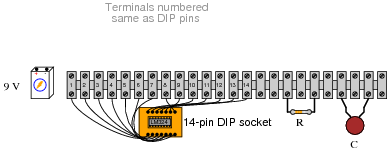The particular opamp used in this circuit is an LM324 (quad) operational amplifier. Of course, you need only show how one of the four opamps within the IC may be connected to form a differentiator.